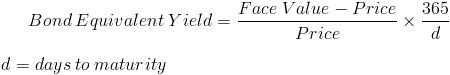# Bond Equivalent YieldThe bond equivalent yield formula is used to determine the annual yield on a discount, or zero coupon, bond. When making investment decisions, comparing the yield or returns on the investment choices in relative terms is important. The return on a 6 month bond would obviously be less than on a 12 month bond, ceteris paribus. Likewise, the percentage of return would be less yet equally profitable when considering the length of investment. The bond equivalent yield formula can be used to compare these two investments with different maturities in relative terms.

## Breakdown of the Bond Equivalent Yield Formula

The first portion of the bond equivalent formula shows the return on investment as a percentage. The face value is the amount paid at maturity and the price is the amount originally paid. By subtracting the price from the face value, the monetary return can be found. In simple terms, if one spends \$75 to be repaid with \$80 in 6 months, that individual has made \$5. By dividing the return by the original price, we can find the percentage return on the investment. Using the prior example of \$5 that was made on the original \$75 purchase, the individual has made 6.667% in those 6 months.

The second portion of the bond equivalent formula annualizes the first portion of the formula. A simple example would be a 6 month bond with a 5% yield during the 6 months. The 5% yield would be determined with the first portion of the formula and multiplying by approximately 2 would give the annual yield. The second portion of the formula for this example would be approximately 2 and not exactly 2 due to there being 365 days in a year which does not exactly fit into a half year.

New to Finance?